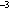U.S. Dept. of Commerce / NOAA / OAR / PMEL / Publications

## 2. CTD data processing

For this study, CTD stations within areal bins in the tropical Pacific are averaged to make a mean hydrographic profile for each bin. In latitude, the bins are centered every 1° from 20°S to 20°N. In longitude, the bins are centered every 5° from 120°E to 75°W. This anisotropy is dictated by the data distribution, consisting mostly of closely sampled meridional sections with wide zonal spacing, but is also appropriate to the tropical ocean interior where zonal scales greatly exceed meridional scales. For this study 15,693 individual CTD stations between 20.5°S and 20.5°N, 120°E and 70°W and taken from 1967 through 1998 are used. These data are all the high-resolution profiles available from the National Oceanographic Data Center (NODC) archives, as well as recent Pacific Marine Environmental Laboratory (PMEL) cruise data and some other cruise data not yet available from NODC. The average profiles are used to make maps of properties on neutral density anomaly,n, surfaces (Jackett and McDougall 1997), meridional-vertical water property sections, and mass transport estimates. Only profiles with more than two CTD casts are used for the property maps and transport estimates and with two or more CTD casts for the vertical sections.

The CTD station data within each bin, consisting of salinity S and temperature T as a function of pressure P are averaged as a function ofn to create mean hydrographic profiles. Averaging S, T, and P as functions ofn is more involved than the conventional approach of averaging S, T, andn as functions of P, but this technique better preserves water properties in the sharp tropical pycnocline and the strength of the pycnocline itself because averaging is quasi-Lagrangian in the vertical (Gouriou and Toole 1993). First, to reduce small-scale noise, individual CTD station S and T profiles are filtered in P with a 5-dbar half-width Hanning filter and subsampled at 5-dbar intervals. Thenn is computed for these subsampled data and averaged as a function of P to obtain a meann(P) profile at 5-dbar resolution. Following this step, the individually filtered and sampled profiles of S, T, and P are linearly interpolated to eachn(P) profile value to allow averaging of the individual CTD station data as a function ofn at roughly 5-dbar resolution. Mean profiles for S(n), T(n), and P(n) are then calculated. Finally the mean P(n) profile is used to put the mean profiles of S(n) and T(n) onto an even 5-dbar grid and construct a final meann profile. The profile is limited to values denser than the densest mixed layer for the CTD stations within the bin.

Depth and properties of the surface mixed layer, includingn, vary over time. To construct a mixed layer for the mean profiles of S(n), T(n), andn, the following procedure is used. First, the mixed layer P for each CTD station is defined as the P above whichn is less than 0.05 kg mdenser than the meann of the top 10 dbar. Mean S and T from the surface to the mixed layer P are calculated for each CTD station. Then these S and T values are averaged to find mean mixed layer values for S and T. These values are used with the mean mixed layer P to calculate a mean mixed layern. In order to avoidn inversions in the mean profiles, the pressure of the mean mixed layern in the final meann profile is used to define a final mean mixed-layer P. To finish, the mean mixed layer S, T, andn values are substituted into the mean S(n), T(n), andn profiles from the mean mixed layer P to the surface. The gap between the mean mixed layer and the mean interior profiles is interpolated using a shape-preserving local spline (Akima 1970).

The analysis exclusively uses the resulting 5-dbar mean hydrographic profiles. The only further vertical smoothing performed is on the square of the buoyancy frequency, N, which is calculated from the mean hydrographic profiles and then smoothed with a 35-dbar median filter before use. The median filter effectively reduces noise while preserving the rapid transition from the surface mixed layer to the pycnocline. This quantity is then used to calculate the planetary component of the potential vorticity, Q = Nf / g. A few Q profiles still have some noise at depth in bins with few stations and some of those ending in shallow water. Neutral surface maps are made by linearly interpolating the 5-dbar values of each mean hydrographic profile to the appropriaten. These values are then objectively mapped assuming a Gaussian covariance with correlation length scales of 10° longitude and 2° latitude and a noise-to-signal variance ratio of 0.25. These correlation length scales are twice the bin size, to emphasize only those large-scale features that are well resolved by the data.